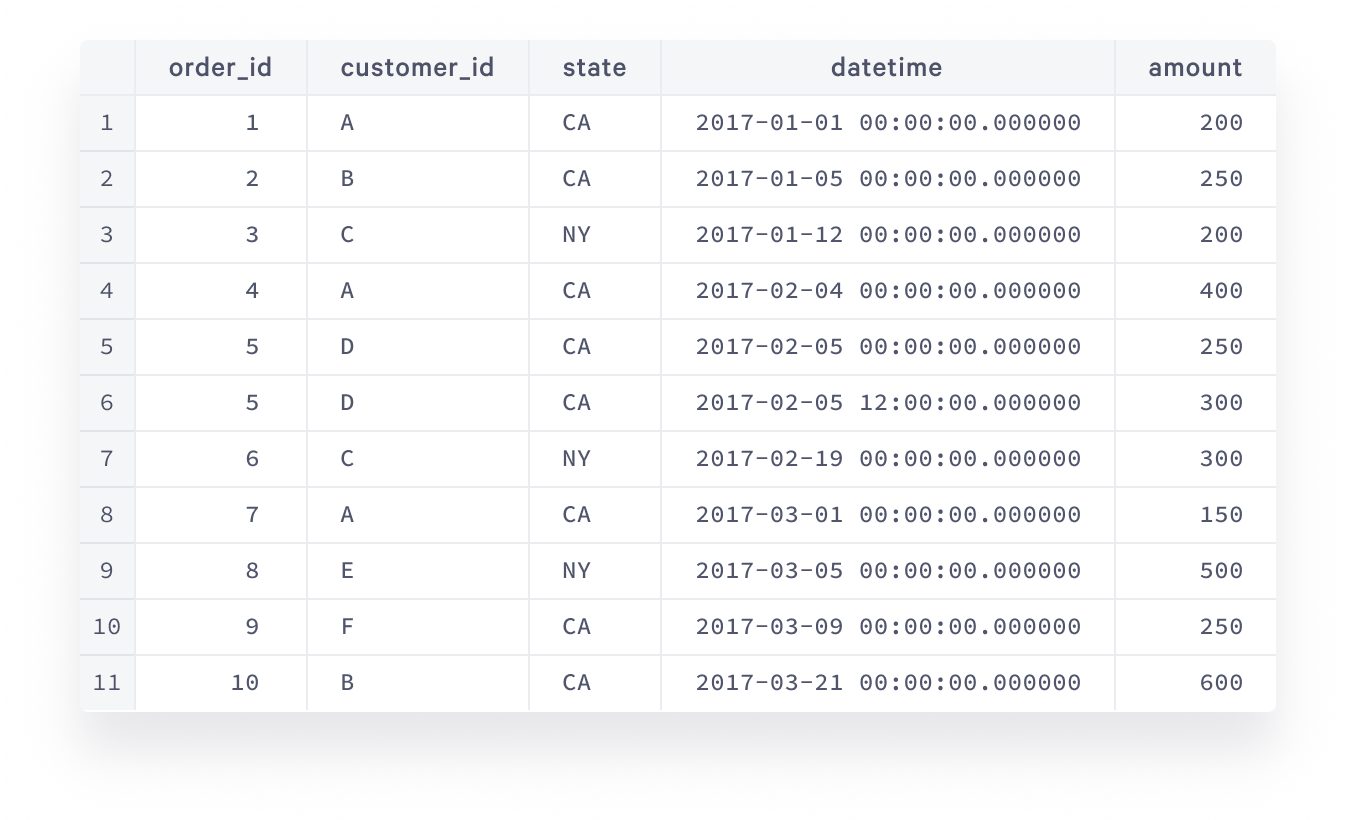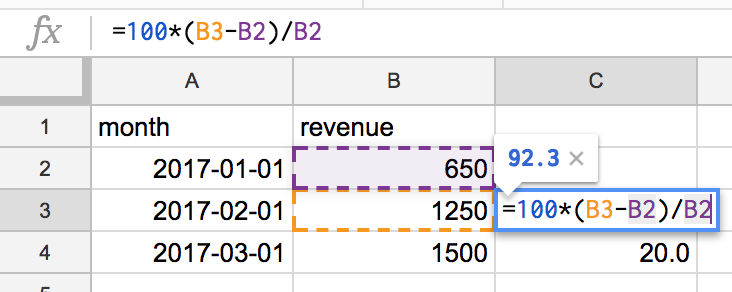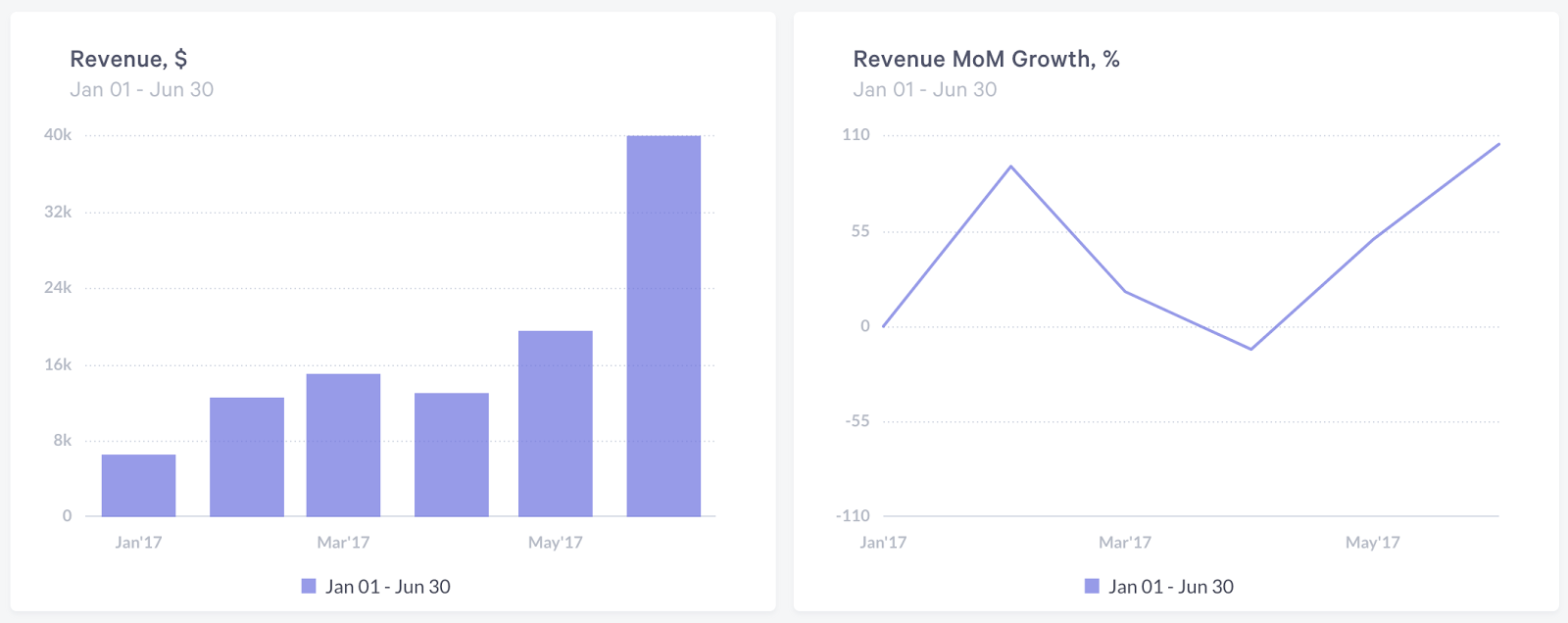# SQL Window Functions Tutorial for Business Analysis

In this SQL window functions tutorial, we will describe how these functions work in general, what is behind their syntax, and show how to answer these questions with pure SQL.comments

By Alex Yeskov, Analytics Lead at ezhome

Any person that has worked with data analytics has had a bad day when they sighed over a problem that was intuitively simple but practically hard to crack using pure SQL.

What is the revenue growth month over month and running total revenue? Can we trust the metric, or does the data have some accidental duplicates that affect it? What are the top N orders for every month? What is the repeat purchase behavior? All these questions have to be translated from business language to programming language.

An intuitive solution in a countless number of cases like these is, “If only I could just loop over the results of my query I would be able to get the answer right away.” This opens a door to a world of workarounds: writing complex joins, data ending up in another spreadsheet, using procedural extensions of SQL, or even moving data processing outside the database. Not all alternatives are viable, others are just ugly.

At the same time, there’s a pure SQL implementation called “window functions” that is very readable, performant, and easy to debug.

In this SQL window functions tutorial, we will describe how these functions work in general, what is behind their syntax, and show how to answer these questions with pure SQL.### Learn by doing

For people unfamiliar with SQL window functions, the official database documentation can be hard to decipher, so we’ll go with real examples, starting from very basic queries and increasing the degree of complexity.

Questions that were listed in the introduction above are real business questions that practically any company faces, and window functions are a huge help with answering them. Imagine we have the following table of orders:Data visualized with Statsbot

This dataset is very simple and small, however it is sufficient to illustrate the power of SQL window functions. Let’s start with the revenue growth.

### Revenue growth

In business terms, revenue growth in month M1 is calculated as:

100*(m1-m0)/m0

where m1 is the revenue in the given month and m0 is the revenue in the previous month. So, technically we would need to find what the revenue is for each month and then somehow relate every month to the previous one to be able to do the calculation above. A very easy way to do this would be to calculate the monthly revenue in SQL:

```SELECT
date_trunc(‘month’,datetime) as month,
sum(amount) as revenue
FROM orders
GROUP BY 1
ORDER BY 1```

then copy the output into the spreadsheet and use a formula to produce a growth metric:When you copy the formula from cell C3 to cell C4 and so on, references are automatically shifted down, that’s what spreadsheets are good at. But what if you’d like to have this metric as a part of a nice dashboard that is fed by data coming directly from the database? Such tools as Statsbot can help you with that:Data visualized with Statsbot

In this case, a spreadsheet is definitely not what you want to end up with. Let’s try to calculate this in SQL. Without window functions, a query that gets you the final result would look like this:

```WITH
monthly_revenue as (
SELECT
date_trunc(‘month’,datetime)::date as month,
sum(amount) as revenue
FROM orders
GROUP BY 1
)
,prev_month_revenue as (
SELECT
t1.*,
t2.revenue as prev_month_revenue
FROM monthly_revenue t1
LEFT JOIN monthly_revenue t2
ON datediff(month,t2.month,t1.month)=1
)
SELECT *,
round(100.0*(revenue-prev_month_revenue)/prev_month_revenue,1) as revenue_growth
FROM prev_month_revenue
ORDER BY 1```

You’d have to calculate monthly revenue, then get the result of the previous month using self-join and use it in the final formula. The logic is broken down into 3 steps for clarity. You can’t break it down further, but even so the second step can be quite confusing.

What you have to keep in mind about datediff is that that the minuend (i.e. what you subtract from) is the third parameter of the function and the subtrahend (i.e. what you subtract) is the second parameter. I personally think that’s a bit counterintuitive for subtraction, and the self join concept itself is not basic. There’s actually a much better way to express the same logic:

```WITH
monthly_revenue as (
SELECT
date_trunc(‘month’,datetime)::date as month,
sum(amount) as revenue
FROM orders
GROUP BY 1
)
,prev_month_revenue as (
SELECT *,
lag(revenue) over (order by month) as prev_month_revenue
FROM monthly_revenue
)
SELECT *,
round(100.0*(revenue-prev_month_revenue)/prev_month_revenue,1) as revenue_growth
FROM prev_month_revenue
ORDER BY 1```

Let’s break down the lag(… line of code:

• lag is a window function that gets you the previous row
• revenue is the expression of what exactly you would like to get from that row
• over (order by month) is your window specification that tells how exactly you would like to sort the rows to identify which row is the previous one (there’s plenty of options). In our case, we told the database to get the previous month’s row for every given month.

This is a generic structure of all SQL window functions: function itself, expression and other parameters, and window specification:

`function (expression, [parameters]) OVER (window specification)`

It is very clean and powerful and has countless opportunities. For example, you can add partition by to your window specification to look at different groups of rows individually:

```WITH
monthly_revenue as (
SELECT
date_trunc(‘month’,datetime)::date as month,
state,
sum(amount) as revenue
FROM orders
GROUP BY 1,2
)
,prev_month_revenue as (
SELECT *,
lag(revenue) over (partition by state order by month) as prev_month_revenue
FROM monthly_revenue
)
SELECT *,
round(100.0*(revenue-prev_month_revenue)/prev_month_revenue,1) as revenue_growth
FROM prev_month_revenue
ORDER BY 2,1```

Calculating revenue by state in the first subquery and updating the window specification to take the new grouping into account, you can look at each state individually and see what the revenue growth by state is.

Partitions are extremely useful when you need to calculate the same metric over different segments.